# Text Feature Extraction in NLPPosted by

### Preface:

In our previous blog, we discussed basic text preprocessing concepts. Whereas in this blog we shall visit methods that are used for text feature extraction.

Every machine/deep learning algorithm takes features as input for its prediction. Therefore after the removal of slag words, we need to convert the text into features.

### Method 1: Number of characters:

Let’s say we have a text, and we want to how many characters are there in the text.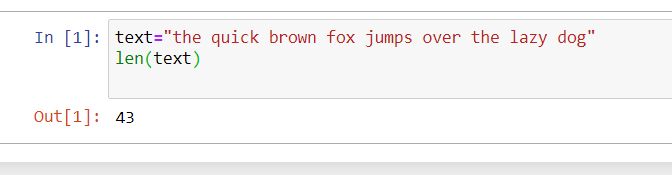### Method 2: Number words in each sentence:

The below code shows how to get the number of words in each sentence. The first sentence has 9 words and the second sentence has 6 words.

Generally on a certain topic, if we like/dislike to a greater extend, we write short comments. In those cases, getting a number of words in a sentence can be helpful.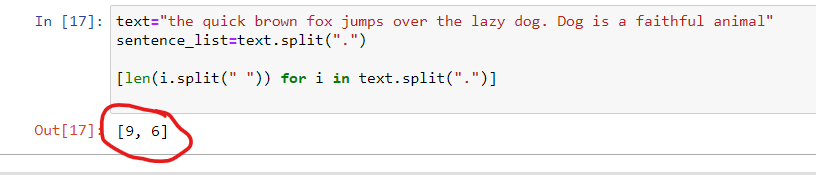### Method 3: Binary encoding and CountVectorizer:

In this method, a text is converted to numeric features. It checks the presence of a word in the given text and how many times it has appeared. We shall use CountVectorizer() of sklearn for this task. CountVectorizer() also converts words into features.we can also convert these features into a table-like structure.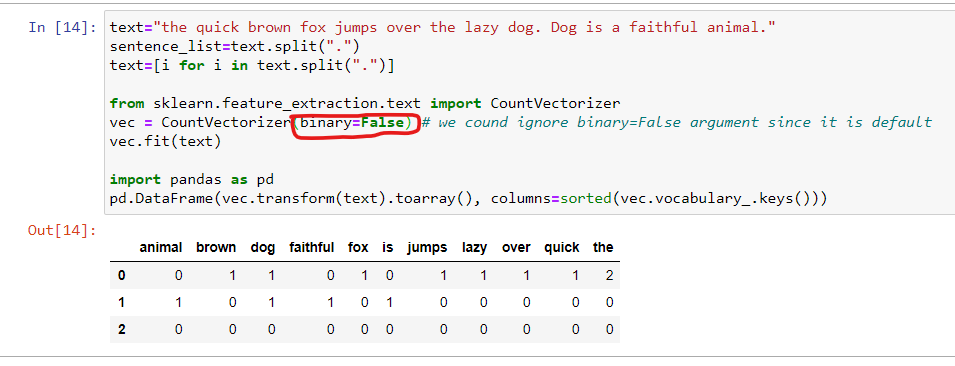If the argument inside CountVectorizer(), binary=False, then it will calculate the number of words in the given text.

### Bag of Words(BOW):

NLP algorithms cannot take raw text directly as input. Therefore, every raw data is converted into a sequence of an array of fixed lengths. BOW is a technique that converts textual data into a real-valued vector. In this method, we make a list of all the unique words and make a local vocabulary. From there we can represent each sentence as a vector, where the presence of each word will be marked as 1 and absence will be marked as 0. Vectorization is the process of converting raw text numerical feature vector. Therefore the result of vectorization is BOW.

##### Sparsity:

In most of the cases, the documents we use are of small size, so a small-sized document has fewer words. Therefore when we convert the documents into vectors, most of the elements are zero.  So the resultant matrix that is created has a large number of zeros.  Hence, we represent the documents in the sparse matrix form.

The pic below shows how to convert a text to a sparsity matrix.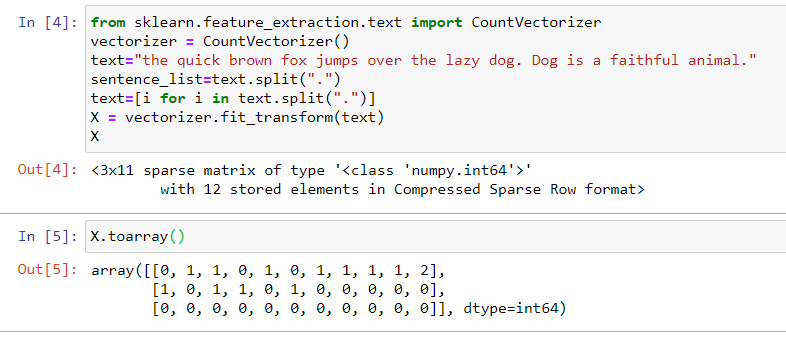### N-grams:

In most layman terms, an n-gram is a pattern of a certain number of words that occurs in the corpus.N-grams is used to predict the next/previous words. Nowadays email providers use n-grams to suggest the probable next word to the user. Let’s say we got a mail where it says, “interview has been postponed”. The email engine automatically generates, “you”, if we type “Thank”. Similarly, it generates, “for the update”, if we type, “thanks”. N-gram also says about the probability of the word that may occur together. Suppose we are calculating the probability of the “w0” occurring after “w1”, then the formula for this is as follows: Count(w1 w0)/count(w1)

The CountVectorizer function of sklearn has a parameter called ngram_range().  It takes two values, which define the lower and upper boundary of the range. For example, ngram_range(1,1) means only unigrams, (1,2) means unigram and bigram, (2,2) means the only bigram.

### Term Frequency-Inverse Document Frequency(TF-IDF):

TF-IDF stands for term frequency-inverse document frequency. Generally, words with high frequency are assigned with a large weight. But there might be cases where high-frequency words might not be important. For example, there is an article about computer science but words like “of”,” is”,” a” are frequent whereas words like “RAM”, “Processor” are less frequent. Therefore even though the occurrence of these is less but they carry high weight with respect to the article. Similarly for words like “destination”,” hotel”,” tour” carry high weight if the article is about tourism. Therefore, TF-IDF assigns more weight to less frequent words based on the corpus.

TF-IDF has two parts:

1. Term frequency(TF): count of the word divided by the total number of words in the document
2. Inverse Document Frequency: responsible for reducing the weights of the words that occur frequently.

IDF can be calculated as log of ( total number of document divided by the number of the document which contains the term)

TF-IDF = TF * IDF.

To make it simple, if a term is very frequent then the IDF value comes near to zero so as TF-IDF inversely if a term is very rare, the IDF value is high.

The code below converts a text into a TF-IDF vector: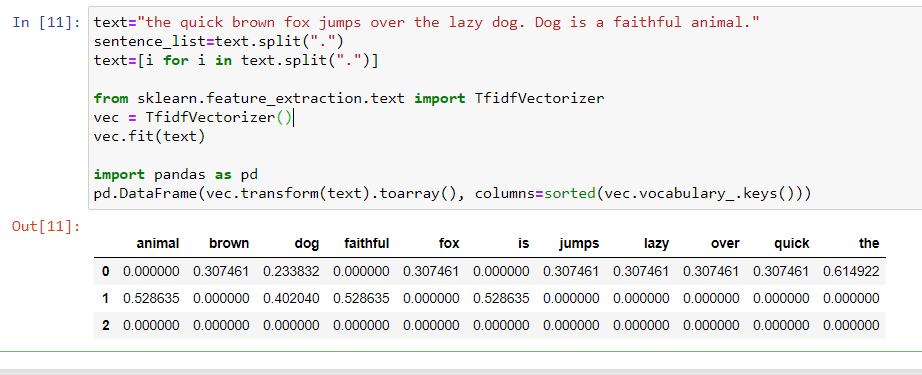### Word Embedding:

Word embedding is a type of word representation in the form of a real value matrix where words of similar meaning are placed in close vicinity of similar words. In other words, it represents words in a coordinate system where every word expressed in a vector-based on a predefined feature set.

### Why word embedding is needed?

There are situations when two different word means the same but for the computer they are different. Let’s consider these two situations. “Pizza tastes great” and “Pizza testes good”. These two statements convey the same meaning. But when we convert these two sentences into one-hot encoding, they are different. Let’s see how.

Step1: take all unique words to make a vocabulary set. X={pizza,testes,good,great}

Step2: convert each word into one-hot encoding.

pizza=[1,0,0,0],testes=[0,1,0,0],good=[0,0,1,0],great=[0,0,0,1].

When we compare these encodings, good and great are different even though they are very similar. One more important aspect is these words are completely independent of each other. Therefore, the ideal model would be, a large matrix where words of similar meaning should be positioned in nearby coordinates.

### Word2Vec:

In Word2Vec, a word is expressed on a high dimensional feature set. Each word is represented by a distribution of weights across those features. In contrast to one-hot encoding, the representation of a word is spread across all the elements in the vector, and each element of the vector contributes to the definition of the word. Word2Vec algorithm, in a nutshell, tries to establish a relationship between the two words. For example, apple minus apples~mango minus mongoes~car minus cars. Therefore using this logic, Word2Vec can be used to answer analogy question, like a man is to woman as uncle is to?

The pic below shows a hypothetical feature vector.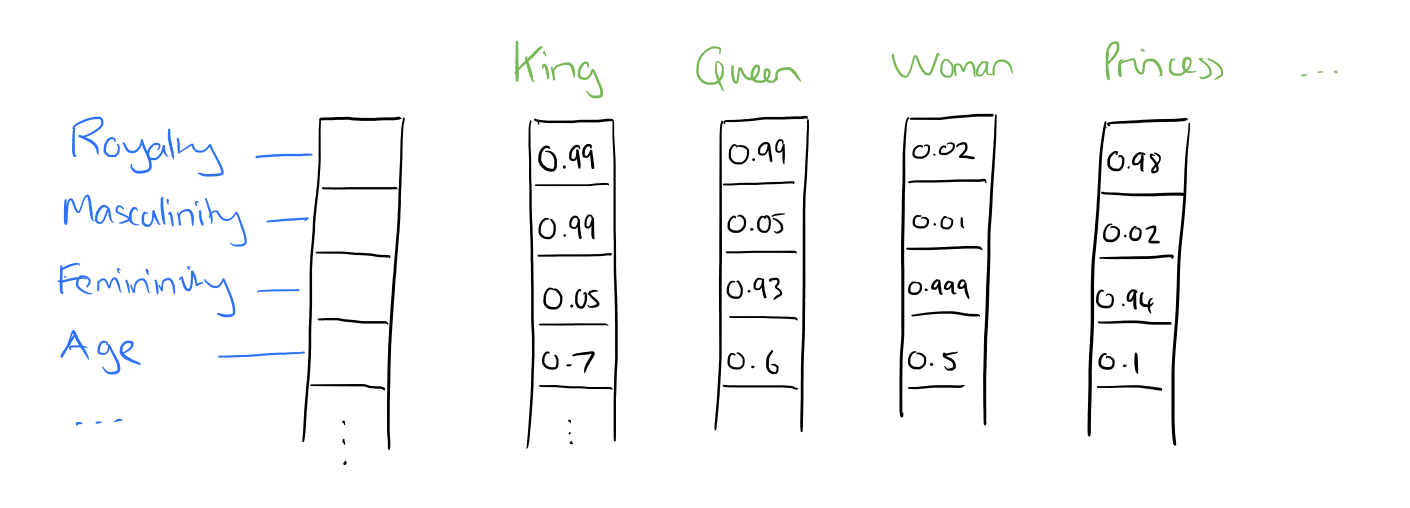From the feature vector, we can infer that words are being expressed based on some parameters. As each word is a vector, we can also execute arithmetic operations, the result of this operation generates a new word. The pic below shows how some of the words are related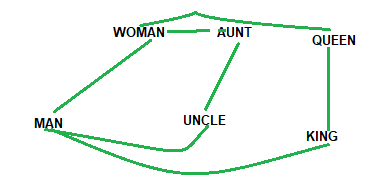Vectors of King, Man, Queen, and Women.

Gensim is a popular open-source library that uses the word2Vec model. It is used for topic modeling and document indexing.

### Glove:

The glove is an unsupervised learning algorithm for deriving vector representation for words. The edge of the Glove vector over Word2Vec is Glove does not just depend on local measures(local context information of the words) but incorporates global statistics to derive word vectors. As Glove considers global measures of a word, it can distinguish the tiny difference between a similar word. For example, “man” and “women”, both words describe human beings. On the other hand, these two words, are opposite since express two opposite gender of human species. In order to show in a quantitative way the variance between “man” and “women”, it is important for a model to relate more than a just single pair of the word.